## 5.19Transient solution

A modern interpretation of the SIMPLE algorithm was described in Sec. 5.12 to couple steady solutions for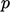and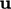. An equivalent transient solution follows an iterative sequence in which equations forandare solved over successive time intervals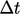, between a start time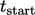and end time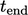.

In a transient simulation,needs to be relatively small to maintain suﬃcient accuracy as the solution evolves over time. The equations do not then generally require under-relaxation to converge due to the increase of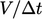to the diagonal coeﬃcients of the matrix from discretisation of the time derivative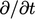.

A transient simulation could follow the same sequence as the SIMPLE algorithm on page 359 , but iterating over that sequence within each time step is costly. A more eﬃcient algorithm solves the sequence only once per time step but adds an iterative loop which substitutes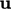from the momentum corrector into the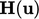term of the pressure equation.

The pressure equation is then solved a second time, followed by a second momentum corrector, in the style of the PISO algorithm.7 The addition of this “PISO loop” improves the accuracy of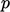,and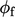within each time step. The improvedbecomes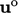in the next time iteration, which critically increases the accuracy of the time derivative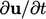in the momentum equation.

Without any iteration over the entire system of equations, the advection terms are discretised using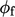from the previous time step. This “lagging” ofdoes not compromise accuracy signiﬁcantly, if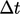is suﬃciently small, e.g. corresponding to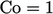.

A further iteration of the PISO loop can be introduced to solve a third pressure equation and momentum corrector, in particular as part of an update to non-orthogonality described in Sec. 5.20 . Increasing the iterations still further is generally not beneﬁcial.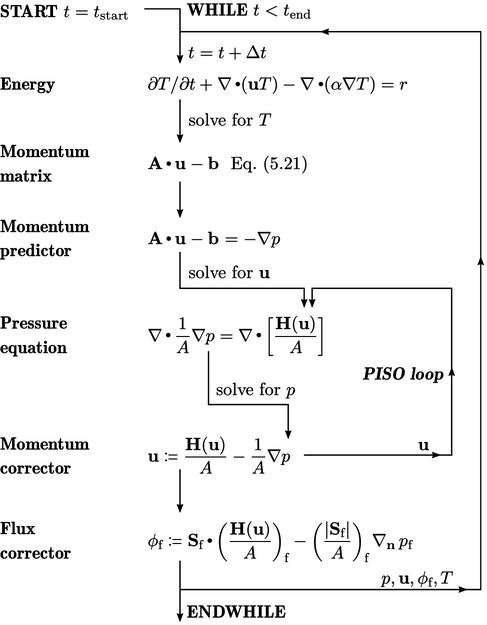7Pressure Implicit with Splitting of Operators, 1986

Notes on CFD: General Principles - 5.19 Transient solution